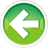- Learn C++ Test Site - https://test.learncpp.com -

# 8.4 — Arithmetic conversions

In lesson 5.1 -- Operator precedence and associativity , we discussed how expressions are evaluated according to the precedence and associativity of their operators.

Consider the following expression:

When binary operator+ is invoked, it is given two operands, both of type `int`. Because both operands are the same type, that type will be used to perform the calculation and to return the result. Thus, `2 + 3` will evaluate to `int` value `5`.

But what happens when the operands of a binary operator are of different types?

In this case, operator+ is being given one operand of type `int` and another of type `double`. Should the result of the operator be returned as an `int`, a `double`, or possibly something else altogether?

In C++, certain operators require that their operands be of the same type. If one of these operators is invoked with operands of different types, one or both of the operands will be implicitly converted to matching types using a set of rules called the usual arithmetic conversions.

The operators that require operands of the same type

The following operators require their operands to be of the same type:

• The binary arithmetic operators: +, -, *, /
• The binary relational operators: <, >, <=, >=, ==, !=
• The binary bitwise arithmetic operators: &, ^, |
• The conditional operator ?: (excluding the condition, which is expected to be of type `bool`)

The usual arithmetic conversion rules

The usual arithmetic conversion rules are pretty simple. The compiler has a prioritized list of types that looks something like this:

• long double (highest)
• double
• float
• unsigned long long
• long long
• unsigned long
• long
• unsigned int
• int (lowest)

There are only two rules:

• If the type of either operand is on the priority list, the operand with lower priority is converted to the type of the operand with higher priority.
• Otherwise (the type of neither operand is on the list), both operands are numerically promoted (see 8.2 -- Floating-point and integral promotion ).

Some examples

In the following examples, we’ll use the `typeid` operator (included in the <typeinfo> header), to show the resulting type of an expression.

First, let’s add an `int` and a `double`:

In this case, the `double` operand has the highest priority, so the lower priority operand (of type `int`) is type converted to `double` value `2.0`. Then `double` values `2.0` and `3.5` are added to produce `double` result `5.5`.

On the author’s machine, this prints:

```double 5.5
```

Note that your compiler may display something slightly different, as the output of `typeid.name()` is left up to the compiler.

Now let’s add two values of type `short`:

Because neither operand appears on the priority list, both operands undergo integral promotion to type `int`. The result of adding two `ints` is an `int`, as you would expect:

```int 9
```

Signed and unsigned issues

This prioritization hierarchy can cause some problematic issues when mixing signed and unsigned values. For example, take a look at the following code:

You might expect the expression `5u - 10` to evaluate to `-5` since `5 - 10` = `-5`. But here’s what actually results:

```unsigned int 4294967291
```

Because the `unsigned int` operand has higher priority, the `int` operand is converted to an `unsigned int`. And since the value `-5` is out of range of an `unsigned int`, we get an result we don’t expect.

Here’s another example showing a counterintuitive result:

While it’s clear to us that `5` is greater than `-3`, when this expression evaluates, `-3` is converted to a large `unsigned int` that is larger than `5`. Thus, the above prints `false` rather than the expected result of `true`.

This is one of the primary reasons to avoid unsigned integers -- when you mix them with signed integers in arithmetic expressions, you’re at risk for unexpected results. And the compiler probably won’t even issue a warning.8.5 -- Explicit type conversion (casting)Index8.3 -- Numeric conversions